# Fractions

Go back to  'Numbers'

## Fractions

Fractions are introduced around grade 3 (typically for students aged 8 or 9). They present a significant challenge for many students. Till now, children have only worked with whole numbers. Suddenly children are shown numbers that have two parts to them. There is a number at the top and another below. Difficult sounding terms like numerator and denominator are introduced. Children only know that each whole number has only one value. Now, two numbers separated by a symbol (½, ¾, etc.) represents a single number adding to the complexity.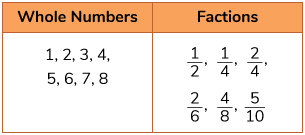## What is a Fraction?

Fractions are a part of a whole.

## Best ways to learn fractions

Visualisation is the best way to learn fractions. Visualisation builds an understanding of fractions as a concept and builds intuition. While teaching fractions emphasis should be on the situations where the object can easily be cut, folded, split or coloured in equal parts. Different manipulatives can also be used which helps in visualising fractions as a part of a whole.

### Using shapesAllow children to compare different visual representations where some part of the figure is shaded. Let children see that a whole has been divided into equal parts and a few of them have been shaded.

### Using strips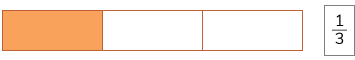Here we represent a strip divided into three equal parts. For the abstract representation, the total number of parts form the denominator. Now, by counting the number of shaded parts, the numerator of the fraction can be obtained.

### Using number line

Ask children to divide the given number line into say three equal parts and then ask the represent each part as fractions. This is extremely helpful as this enables children to see that fractions are numbers, which can be placed on the number line.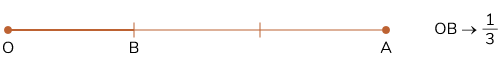## Topics closely related to fractions

The chart given below shows the topics that are a pre-requisite to understanding fractions. It also shows topics that are related to fractions.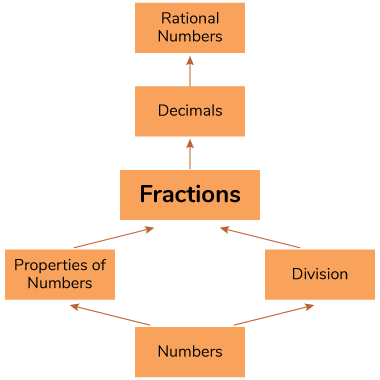## Simple ways to learn fractions

Here are a few time-tested and effective ways that you can use to help your child out at home:

### Practice and more practice:

After understanding the concept then keep practising it. Nothing helps children learn faster than applying the concepts that they are taught. Research has shown that it allows them to cement their concepts and it leads to longer retention.

Clear the common doubts that children usually have while comparing, ordering or operating on fractions.

1. Clear doubts such as when comparing fractions, one can only compare like fractions. Explain to the child this is primarily because fractions represent parts of the same whole, so we utilize the concepts of Equivalent Fractions to make them comparable.## Common mistakes or misconceptions

Misconception 1: Counting the shaded part is enough to identify the fraction.

Explanation: To identify a fraction, all parts must be equal.For a part to be $$\frac{1}{4}$$th, 4 such parts should add up to make a whole. But if some parts are smaller, then depending on the shape we take the whole will be different.

Misconception 2: When finding fraction using area models, children incorrectly believe that the equal sized-piece must also look the same.Children say this diagram does not show $$\frac{1}{2}$$ of the area of the square coloured because the pieces are “not the same (shape)”. They should know that if the areas of the parts are equal then they are identical. To address this use different geometrical shapes like circle, square, etc. and show how differently these shapes can be divided into the same number of parts.

Misconception 3: Children do not realise that while defined as parts of the whole, fractions are numbers. Children fail to understand that $$\frac{1}{2}$$, $$\frac{1}{4}$$, etc. are also numbers. To address this, use the number line model to show the children how fractions exist between whole numbers and how even whole numbers can be denoted using a fractional form. For instance, show 5/8 the following number line. Stress on how $$\frac{5}{8}$$ and $$\frac{7}{8}$$ lie between 0 and 1 as $$\frac{8}{8}$$ is nothing but 1 on the number line written in fractional form since the numbers between 0 and 1 are divided into 8 parts here.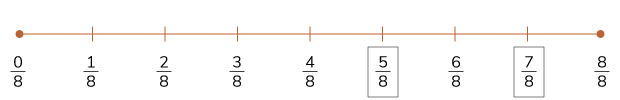Misconception 4: All fractions are less than 1. So, children may incorrectly believe that fractions are always less than 1. This will create a barrier to understand improper fractions. While it may be okay to not address this when fractions are just being introduced, do watch out for this misconception as children build fluency.

## Tips and Tricks

1. Every time there’s an opportunity to talk about fractions, make use of it. When you open a pack of biscuits, ask children what fraction of the whole packet does each biscuit represent. When eating a pizza or cutting a cake or fruits this exercise can be repeated.

2. Referring to fractions such as $$\frac{3}{4}$$ as “three out of four” in addition to “three fourths” helps reiterate the idea that this fraction indicates 3 parts out of 4. Get students familiar with reading fractions in both these ways.

3. Don't introduce terms like numerator and denominator till students are completely comfortable with describing fractions in a simpler language like “three out of four”.

## FAQ

Q1. Why $$3\frac14$$ is called a mixed fraction?

A whole number, when combined with a fraction, is known as a mixed fraction. Taking the above example is the whole number combined with a fraction $$\frac14$$.

## Important Topics

Here are a few links that will take you through the journey that every Cuemath students undertake in the pursuit of understanding Fractions along with practice worksheets:

More Important Topics
Numbers
Algebra
Geometry
Measurement
Money
Data
Trigonometry
Calculus